# Solution assignment 08 Addition and subtraction formulas

### Assignment 8

A point moves in the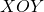-plane. It moves in the-direction according to the function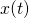and in the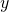-direction according to the function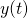. When we have: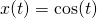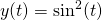calculate the equation of the curve along which the point is moving.

### Solution

We know that we can write for: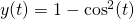Because we have:we can write: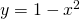This is an 'opens up' parabola with top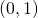. The domain is: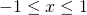and the range: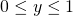0